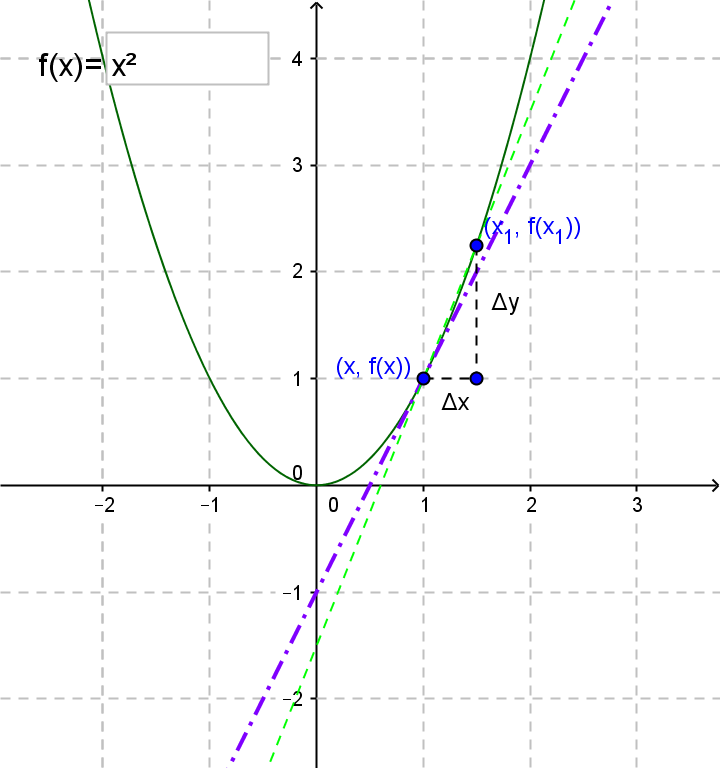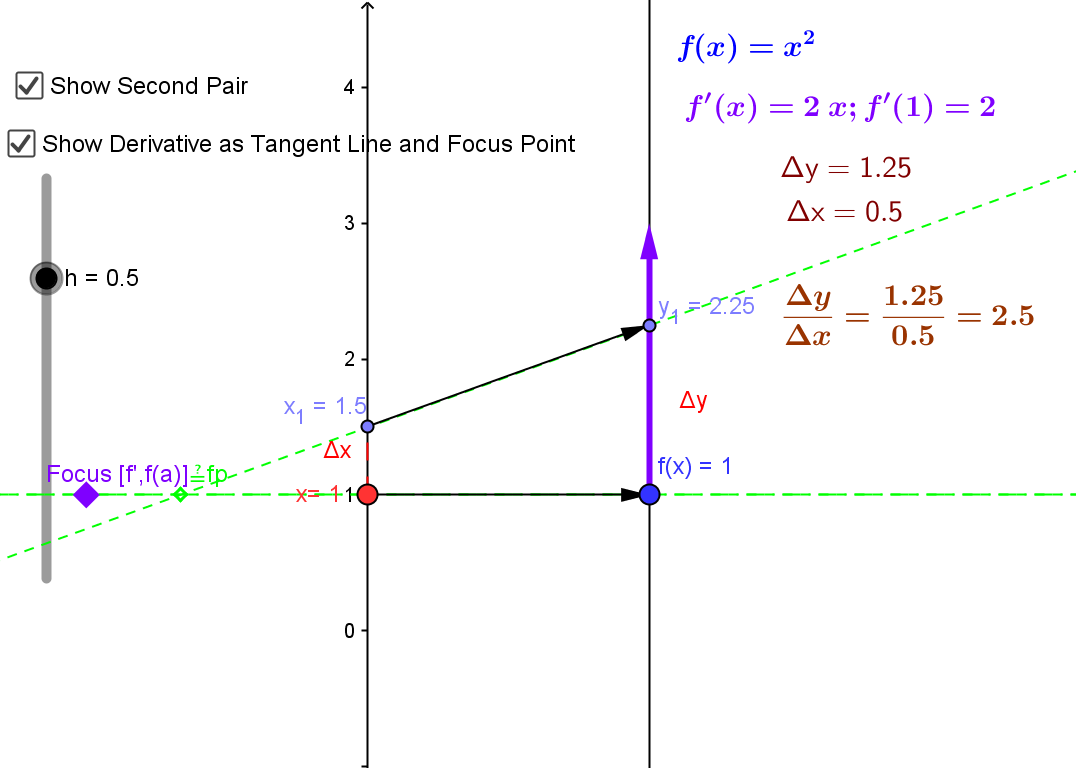The  derivative of $f$ at $a$ is a number, denoted $f'(a)$, which is defined by  $$f'(a) = \lim_{x \rightarrow a} \frac {f(x)-f(a)}{x-a}$$ or  $$f'(a) = \lim_{h \rightarrow 0} \frac {f(a+h)-f(a)}{h}.$$
The ratio used in the
limit expressions are interpreted
1. The average velocity for the time interval determined by a fixed time and a varying instant for an object moving on a straight line;
2. The slope of a secant line between a fixed point and a varying point on the graph of the function;
3. The average rate of change of a variable depending on an independent variable determined by a fixed and a varying value of the independent variable.The derivative can be visualized using a tangent line on a graph or a focus point and derivative "vector" on a mapping diagram.

Martin Flashman. Created with GeoGebra

On the mapping diagram the derivative can be visualized using focus points for the average rates that converge to a single focus point for the best linear approximating function.
The derivative can also be visualized as a vector on the target of the mapping diagram.